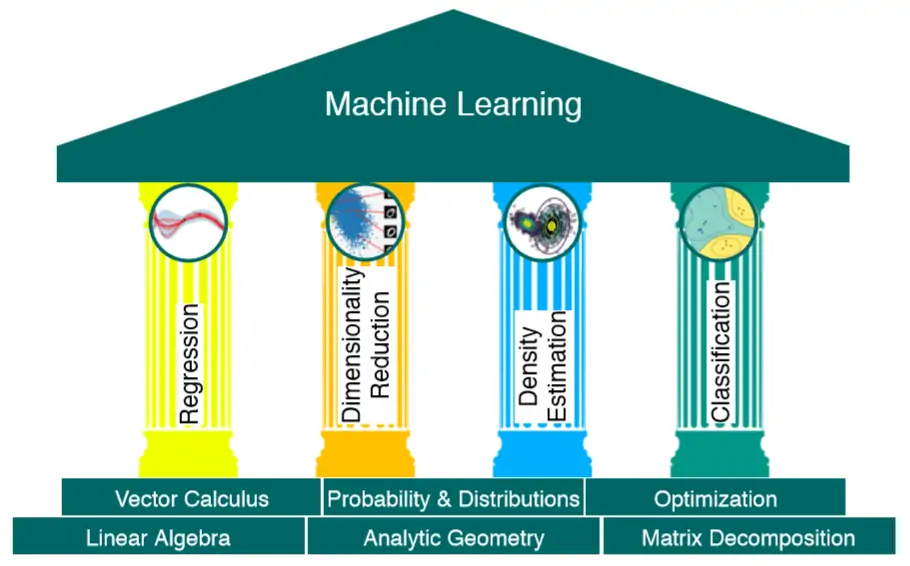Part of the Machina blogchain.

## Background

This post, and several subsequent posts in this blogchain, provides an overview of my learnings derived from the book ‘Mathematics for Machine Learning’—a free book available online here.

After spending some time looking at machine learning applications, I realised I do not yet possess the mathematical framework required to fully grasp the concepts. I am currently able to ‘fail forward’ in a sense; undertaking a project and troubleshooting each step as I go. Fundamentally, however, I felt that I was missing a good chunk of understanding that comes with the intelligent application of theory to solve problems.

Perhaps counterintuitively, then, I am stepping back to move forward. This book is perfectly positioned to serve as an excellent introduction to the core skills of machine learning. From the book’s foreword:

Current machine learning textbooks primarily focus on machine learning algorithms and methodologies and assume that the reader is competent in mathematics and statistics. Therefore, these books only spend one or two chapters of background mathematics, either at the beginning of the book or as appendices. We have found many people who want to delve into the foundations of basic machine learning methods who struggle with the mathematical knowledge required to read a machine learning textbook. Having taught undergraduate and graduate courses at universities, we ﬁnd that the gap between high school mathematics and the mathematics level required to read a standard machine learning textbook is too big for many people. This book brings the mathematical foundations of basic machine learning concepts to the fore and collects the information in a single place so that this skills gap is narrowed or even closed.

## Introduction and Motivation

• The goal of machine learning is to design general-purpose methodologies to extract valuable patterns from data, ideally without much domain-speciﬁc expertise.
• To achieve this goal, we design models that are typically related to the process that generates data, similar to the dataset we are given.
• Learning can be understood as a way to automatically ﬁnd patterns and structure in data by optimizing the parameters of the model.

A model is said to learn from data if its performance on a given task improves after the data is taken into account. The goal is to ﬁnd good models that generalize well to yet unseen data, which we may care about in the future.

To summarise the main concepts of machine learning that are covered in this book:

1. We represent data as vectors.
2. We choose an appropriate model, either using the probabilistic or optimization view.
3. We learn from available data by using numerical optimization methods with the aim that the model performs well on data not used for training.

By way of providing further context, below are the key topics/areas which are outlined in the book and will subsequently be recorded in this blogchain:

• Part 1: Mathematical Foundations
• Linear Algebra
• Analytic Geometry
• Matrix Decompositions
• Vector Calculus
• Probability and Distributions
• Continuous Optimisation
• Part 2: Central Machine Learning Problems
• When Models Meet Data
• Linear Regression
• Dimensionality Reduction with Principal Component Analysis
• Density Estimation with Gaussian Mixture Models
• Classification with Support Vector Machines

The topics of Part 1 are closely interrelated with the problems in Part 2, as illustrated by the diagram below:The Pillars of Machine Learning

At this stage, I am not certain to what level this blogchain will detail these topics. With such heavy mathematical content and symbol density, typing out proofs or examples would prove to be laborious and counter-intuitive but I do hope to be able to share some key insights, connect the dots or generally share the process with others—as well as more personal details like how I am coping in terms of complexity and how I am approaching the study aspect of this project.